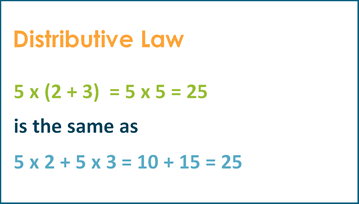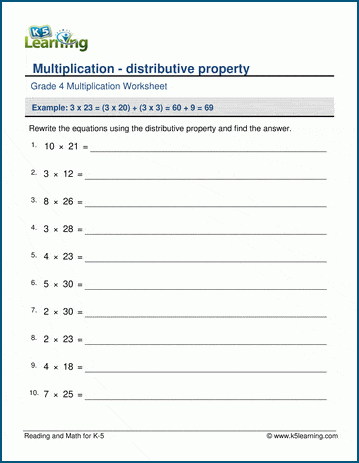# Distributive Law in Math

## What is distributive law?

Distributive law, also called distributive property, is a law relating to the operations of multiplication and addition, which states:

a x (b + C) = a x b + a x c

Distributive law shows that adding several numbers and then multiplying the sum by a number is the same as first multiplying each separately by the number and then adding the products.

5 x (2 + 3)  = 5 x 5 = 25

Is the same as

5 x 2 + 5 x 3 = 10 + 15 = 25## Distributive property worksheets

In our grade 4 math section we have a set of worksheets where students can practice using the distributive law.Become a Member

This content is available to members only.